# Free Vibration of a Spring Mass System

1.7.1 Virtual Experimentation Procedure

Objective

To find out the natural frequency of a spring-mass system and verifying the result with the theoretically obtained natural frequency.

This page describes the main objective of the experiment and also experimental setup was shown (see Fig. 1.12). Click on the next button to proceed further. If the button does not work we request the user to go for the tabs of the respective pages.

###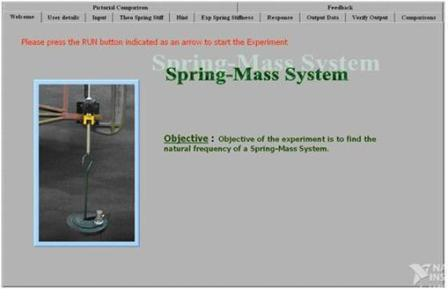Step 2. User Info
The information of the user, doing the experiment will be taken here (it is not compulsory). There will be some basic questions on the experiment. User must pass the test with a minimum score of 1 to continue the experiment. If could not pass the test, we request the user to go through the documentation again and re-start the experiment (see Fig. 1.13).

###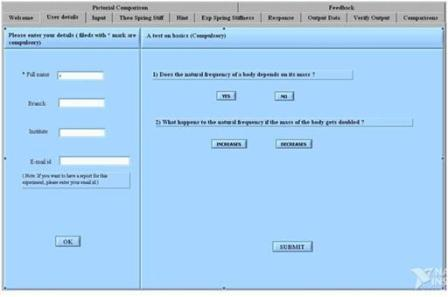Fig. 1.13: User details page

Step 3. Input Page
Now select a combination of spring and mass system to perform experiment and to calculate the natural frequency of that system. There are totally 4 combinations of spring and mass to do the experiment. Because of the limitations we had while doing the experiment, the selection of spring and mass is restricted to particular combinations only (see Fig. 1.14).

###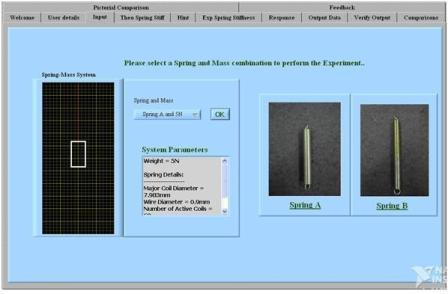Fig. 1.14 User input page

Step 4. Theoretical Spring Stiffness -
Using the strength of materials approach try to find the spring stiffness and with that find out natural frequency of the system (See Fig. 1.15). Go to next page to find some hint for the same and check your answer there by using the SOLUTION button (see Fig. 1.16).

###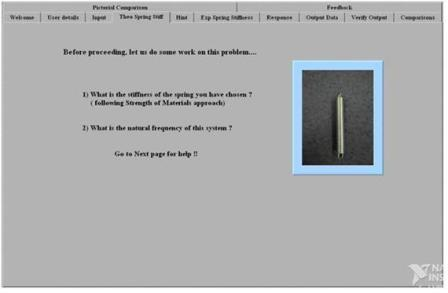Fig. 1.15: Finding the stiffness of the spring theoretically

###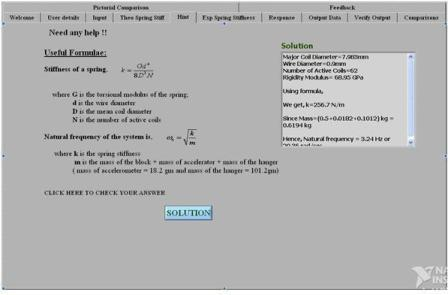Fig. 1.16: Solution page for the spring stiffness

Step 5. Experimental Spring Stiffness -
This page shows a plot of load vs deflection data we got during the experiment. The slope of the plot indicates the stiffness of the corresponding spring. Using that, the natural frequency of the system is calculated and is shown here (see Fig. 1.17).

###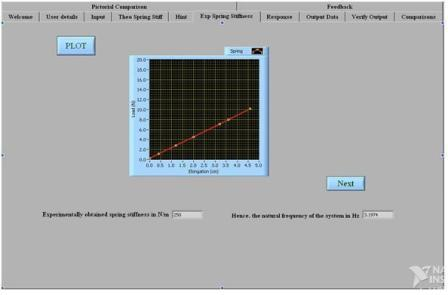Fig. 1.17: Finding spring stiffness, experimentally

Step 6. Response -
This page shows plots with the data obtained during the free vibration of the particular spring-mass system, chosen by you at the input page. This contains two plots and an animation of the vibration movements of the system. One of the plots is the response plot and its FFT plot. Vibrations from the system will decay out with time. Hence, we get a gradual decaying curve, which is used to find the damping factor and the natural frequency of the system using the formulae mentioned in section 1.5 (see Fig. 1.18). The database contains 10 experimental files for each combination of spring and mass setup. Stopping the simulation in the middle and starting the simulation again will retrieve other file from the database. User will get a feel of doing the experiment in real, because, one may carry out the experiment for several trials.

###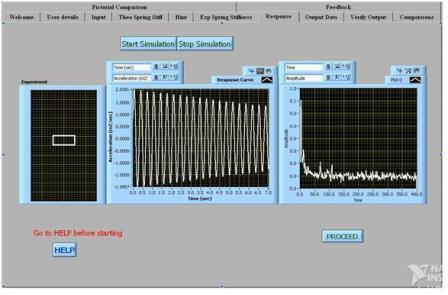Fig. 1.18: Response data

Step 7. Output Data -
This gives the time periods corresponding to each cycle of the response curve. Using this data and the formulae mentioned in section 1.5, find out the logarithmic decrement, damping factor and the natural frequency of the system following the hints provided over there (see Fig. 1.19).

###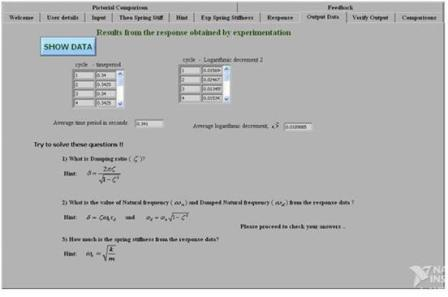Fig. 1.19: Output data from response

Step 8. Verify Output -
Verify your results with the results in Verify Output page (see Fig. 1.20).

###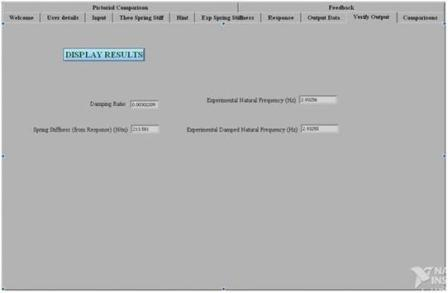Fig. 1.20: Results from the response obtained

Step 9.  Comparisons -
Comparison of results obtained from theoretical, static and dynamic response data are compared here and the conclusion for those results are given. If you want to perform the experiment with other combination of spring and mass, so that you can compare the results of two setups, click on YES button (see Fig. 1.21) and repeat the experiment.

###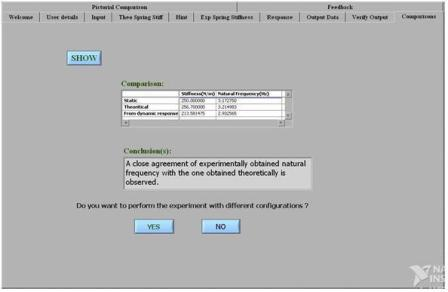Fig. 1.21: Numerical comparison of results

Step10. Pictorial Comparison -
This pages gives the pictorial view of comparisons displayed in the previous page (see Fig. 1.22).

###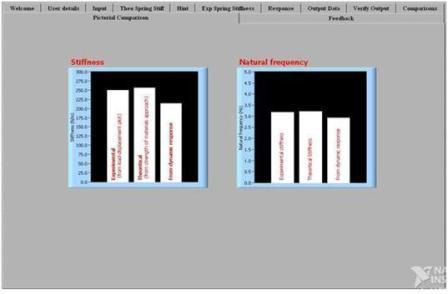Fig. 1.22: Pictorial comparison of results

Step 11. Feedback
Please give your feedback and suggestions on this experiment. This will be saved in our server, so that we can improve our experiment based on your feedback (see Fig. 1.23).

###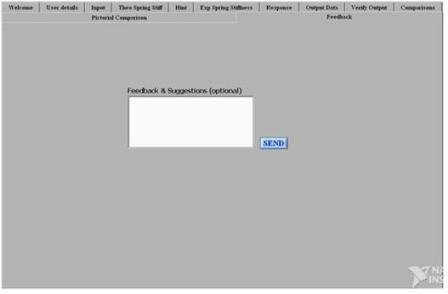Fig. 1.23: Feedback page and end of the experiment